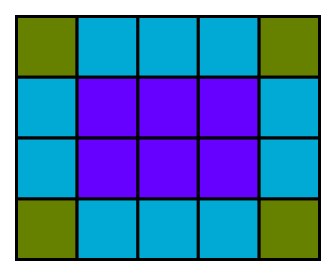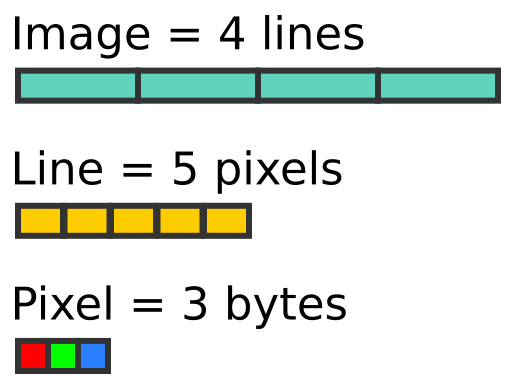# Image processing with numpy

Martin McBride, 2021-09-21
Tags image processing rgb transparency
Categories numpy pillowIn this section, we will learn how to use NumPy to store and manipulate image data. We will use the Python Imaging Library (PIL) to read and write data to standard file formats.

This article explains how image data is stored in a NumPy array. Other articles include:

Before trying these examples you will need to install the NumPy and Pillow packages (Pillow is a fork of the PIL library).

## Creating RGB Images

Here is a 5 by 4 pixel RGB image:The image contains 4 lines of pixels. Each line of pixels contains 5 pixels. Each pixel contains 3 bytes (representing the red, green and blue values of the pixel colour):RGB images are usually stored as 3-dimensional arrays of 8-bit unsigned integers. The shape of the array is:

```height x width x 3.
```

Here is how we create an array to represent a 5 pixel wide by 4 pixel high image:

```import numpy as np

width = 5
height = 4

array = np.zeros([height, width, 3], dtype=np.uint8)
```

Notice that the first dimension is the height, and the second dimension is the width. That is because the data is ordered by lines, then each line is ordered by pixels, and finally, each pixel contains 3-byte values for RGB. Each colour is represented by an unsigned byte (`numpy` type `uint8`).

Now let's fill the array with orange pixels (red=255, green=128, blue=0). We use slices to do this, the three values are broadcast across all the rows and columns of the array:

```array[:,:] = [255, 128, 0]
```

## Saving an RGB image using PIL

Now we can use `fromarray` to create a PIL image from the NumPy array, and save it as a PNG file:

```from PIL import Image

img = Image.fromarray(array)
img.save('testrgb.png')
```

In the code below we will:

• Create a 200 by 100 pixel array
• Use slice notation to fill the left half of the array with orange
• Use slice notation to fill the right half of the array with blue

Here is the complete code:

```import numpy as np
from PIL import Image

array = np.zeros([100, 200, 3], dtype=np.uint8)
array[:,:100] = [255, 128, 0] #Orange left side
array[:,100:] = [0, 0, 255]   #Blue right side

img = Image.fromarray(array)
img.save('testrgb.png')
```

And here is the image:## Creating RGBA images

An RGBA image has 4 channels (unlike an RGB image that has only 3). The fourth channel is an alpha channel. An alpha value of 255 will make the pixel fully opaque, value 0 will make it fully transparent, values in between will make the pixel partly transparent.

In the code below we create an RGBA image, initially setting the same blue and orange areas as before, with an alpha value of 255. We then loop over the image changing the alpha value of each pixel to be equal to its `x` coordinate. This means that the pixels on the left side of the image will be transparent, and the pixels at the right will be almost fully opaque. The transparency varies smoothly from left to right.:

```import numpy as np
from PIL import Image

array = np.zeros([100, 200, 4], dtype=np.uint8)
array[:,:100] = [255, 128, 0, 255] #Orange left side
array[:,100:] = [0, 0, 255, 255]   #Blue right side

# Set transparency depending on x position
for x in range(200):
for y in range(100):
array[y, x, 3] = x

img = Image.fromarray(array)
img.save('testrgba.png')
```

And here is the image:## Creating greyscale images

Greyscale images are handled slightly differently. Because there is only one channel, there is no need to create a 3-dimensional array, you should use a 2-dimensional array instead:

```import numpy as np
from PIL import Image

array = np.zeros([100, 200], dtype=np.uint8)

# Set grey value to black or white depending on x position
for x in range(200):
for y in range(100):
if (x % 16) // 8 == (y % 16) // 8:
array[y, x] = 0
else:
array[y, x] = 255

img = Image.fromarray(array)
img.save('testgrey.png')
```

In this case, we have created a chequerboard image:You can read an image using the PIL `open` function, and convert it to an array using the numpy `array` function. Here, we read the images that were created previously, and print their NumPy shape:

```import numpy as np
from PIL import Image

img = Image.open('testrgba.png')
array = np.array(img)
print(array.shape)      # (100, 200, 4)

img = Image.open('testrgb.png')
array = np.array(img)
print(array.shape)      # (100, 200, 3)

img = Image.open('testgrey.png')
array = np.array(img)
print(array.shape)      # (100, 200)
```

You can manipulate the image data and write it back out to a file. For example, this code inverts a greyscale image (swapping black and white). and saves it back:

```import numpy as np
from PIL import Image

img = Image.open('testgrey.png')
array = np.array(img)

array = 255 - array

invimg = Image.fromarray(array)
invimg.save('testgrey-inverted.png')
```

Visit the PythonInformer Discussion Forum for numeric Python.

If you found this article useful, you might be interested in the book NumPy Recipes or other books by the same author.

#### Popular tags

2d arrays abstract data type alignment and animation arc array arrays bezier curve built-in function callable object circle classes close closure cmyk colour comparison operator comprehension context context manager conversion creational pattern data types design pattern device space dictionary drawing duck typing efficiency else encryption enumerate fill filter font font style for loop function function composition function plot functools game development generativepy tutorial generator geometry gif gradient greyscale higher order function hsl html image image processing imagesurface immutable object index inner function input installing iter iterable iterator itertools l system lambda function len line linspace list list comprehension logical operator lru_cache magic method mandelbrot mandelbrot set map monad mutability named parameter numeric python numpy object open operator optional parameter or partial application path polygon positional parameter print pure function pycairo radial gradient range recipes rectangle recursion reduce rgb rotation scaling sector segment sequence singleton slice slicing sound spirograph sprite square str stream string stroke subpath symmetric encryption template text text metrics tinkerbell fractal transform translation transparency tuple turtle unpacking user space vectorisation webserver website while loop zip